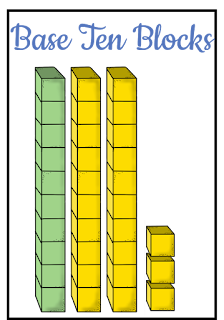# Adding and Subtracting Ten Mentally

For some first grade students, adding and subtracting ten to a given number comes naturally. They know that 23 has 2 tens and 3 ones so, naturally adding another ten gives the answer of 33! For other students, this skill isn’t so intuitive. You may have taught the skill using a place value chart and, while 80% of the class picked it up using that representation, there are still a few students who will tell you, with confidence, that 23 + 10 = 24.A place value chart model for adding ten.

I have written at length about the C-R-A framework of teaching math. When I think about the skill and understanding required to add or subtract ten and I think about using a place value chart to promote understanding I see that the reason why some kids are falling out behind the rest of the class is that a place value chart alone is a representative (verging on abstract) model. What about the students who needed to take this skill back to the concrete level?

A strong method for teaching addition and subtraction of 10 is to start with the concrete, link it to a representation and then move to a more abstract model. Read on to see how each of these steps play out in the classroom:

ConcreteI have a few preferred concrete models for teaching this skill. First, I love using base ten blocks . They are an accessible tool in the classroom, students likely have already gained familiarity with the tool and they adequately demonstrate the difference in magnitude between a one and a ten. When teaching this skill I ask students to build a number with ten sticks and ones. I then ask them to tell me about what block they would need to have to show ten more. We may have some discussion about It is so important that students can articulate that when they added a ten the ones remained the same but the tens changed. If they aren’t noticing this pattern, help them to notice and then test the pattern. Without this understanding, they will have a very hard time generalizing the rule!

how we could use either a ten stick or ten ones but ultimately, push them to use a ten stick as it is the more efficient way of counting. Students then add a ten stick to their original number and write an equation to demonstrate what happened. For example: 23 + 10 = 33. I then ask students to tell me what happened to the tens and what happened to the ones using place value language.

You can also use \$10 bills and \$1 bills to model this relationship. If your students are very strong in coin names and values you can also use dimes an pennies to demonstrate this relationship. If your students aren’t super solid in their understanding of coins, I would save that representation for another day. I am a huge proponent of using dimes and pennies to teach and reinforce place value concepts, however, I am a bigger proponent of staying focused and I don’t want to muddy the waters in terms of the focus of this lesson.

Representative

As mentioned above, a strong model for representing the number of tens and ones before and afterten is added or subtracted would be a place value chart. If your students are demonstrating that they are able to use concrete materials but that the place value chart is a bit of a reach for them at this time, consider having your students draw a representation of ten sticks and ones. They can do this parallel to their use of base ten blocks and then drop the manipulatives once they are able to perform the skill AND talk about the problem using base ten language using their drawing alone.

Abstract

Just because you are teaching using the CRA framework does not mean that you are teaching each of these three skills in isolation. As I mentioned in the “Representative” paragraph, the best way into the representative model is to ask students to do so parallel to the “Concrete” model so that students can make explicit connections. The abstract model of this work being, for example, a number sentence, can be linked to both the representative and the abstract. I ask students on day 1 of this skill when they are building with blocks to write a number sentence that matches what they have built with blocks. As students are drawing place value drawings I also ask them to write an equation that represents what they have done.

And always, always, always, promote math talk and math language when your students are working. Their ability to articulate their thinking and the math processes will help to solidify their understanding and build connections to future learning!

If you are looking for a math resource that will better guide you and your students through the development of this skill (1.NBT.5) from concrete to mental math please take a look at my 5 Day Focus: 10 More and 10 Less. This resource includes a pre and post assessment, 5 days of detailed lesson plans, independent, hands on activities for each day and tickets out the door for each lesson.